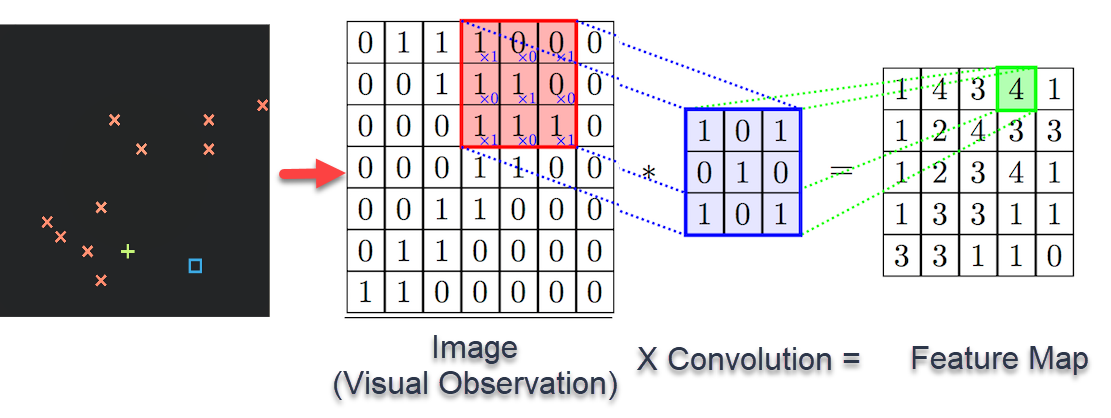# Convolutional Neural Network (CNN) in a brief

### What is Convolutional Neural Network (CNN) ?

• A neural network in which at least one layer is a convolutional layer.
• Depending on features, we categorize the images (classify) using CNN.
• Yann Lecun is considered the grandfather of Convolutional neural networks.

### What is a Convolutional Layer ?

These are the layers of convolutional neural network where filters are applied to the original image.

### Steps involved in constructing a Convolutional Neural Network:

1. Convolution Operation.
2. Stride.
3. ReLU Layer.
4. Pooling.
5. Flattening.
6. Full Connection.

### 1. Convolution Operation :

• In this process, we reduce the size of the image by passing the input image through a Feature detector/Filter/Kernel so as to convert it into a Feature Map/ Convolved feature/ Activation Map
• It helps remove the unnecessary details from the image.
• We can create many feature maps (detects certain features from the image) to obtain our first convolution layer.
• Involves element-wise multiplication of convolutional filter with the slice of an input matrix and finally the summation of all values in the resulting matrix.Fig 2. Convolution Operation on a matrix / Image

#### 1.1. Stride:

The number of pixels by which we are moving the filter over the input matrix is called a stride.

#### 1.2. ReLU Activation Function :

• ReLU is the most commonly used activation function in the world.
• When applying convolution, there is a risk we might create something linear and there we need to break linearity.
• Rectified Linear unit can be described by the function f(x) = max(x, 0).
• We are applying the rectifier to increase the non-linearity in our image/CNN. Rectifier keeps only non-negative values of an image.

### 2. Pooling :

• It helps to reduce the spatial size of the convolved feature which in-turn helps to to decrease the computational power required to process the data.
• Here we are able to preserve the dominant features, thus helping in the process of effectively training the model.
• Converts the Feature Map into a Pooled Feature Map.

Pooling is divided into 2 types: 1. Max Pooling - Returns the max value from the portion of the image covered by the kernel. 2. Average Pooling - Returns the average of all values from the portion of the image covered by the kernel.

### 3. Flattening :

Involves converting a Pooled feature Map into one-dimensional Column vector.

### 4. Full Connection :

• The flattened output is fed to a feed-forward neural network with backpropagation applied to every iteration.
• Over a series of epochs, the model is able to identify dominating features and low-level features in images and classify them using the Softmax Classification technique (It brings the output values between 0 and 1).